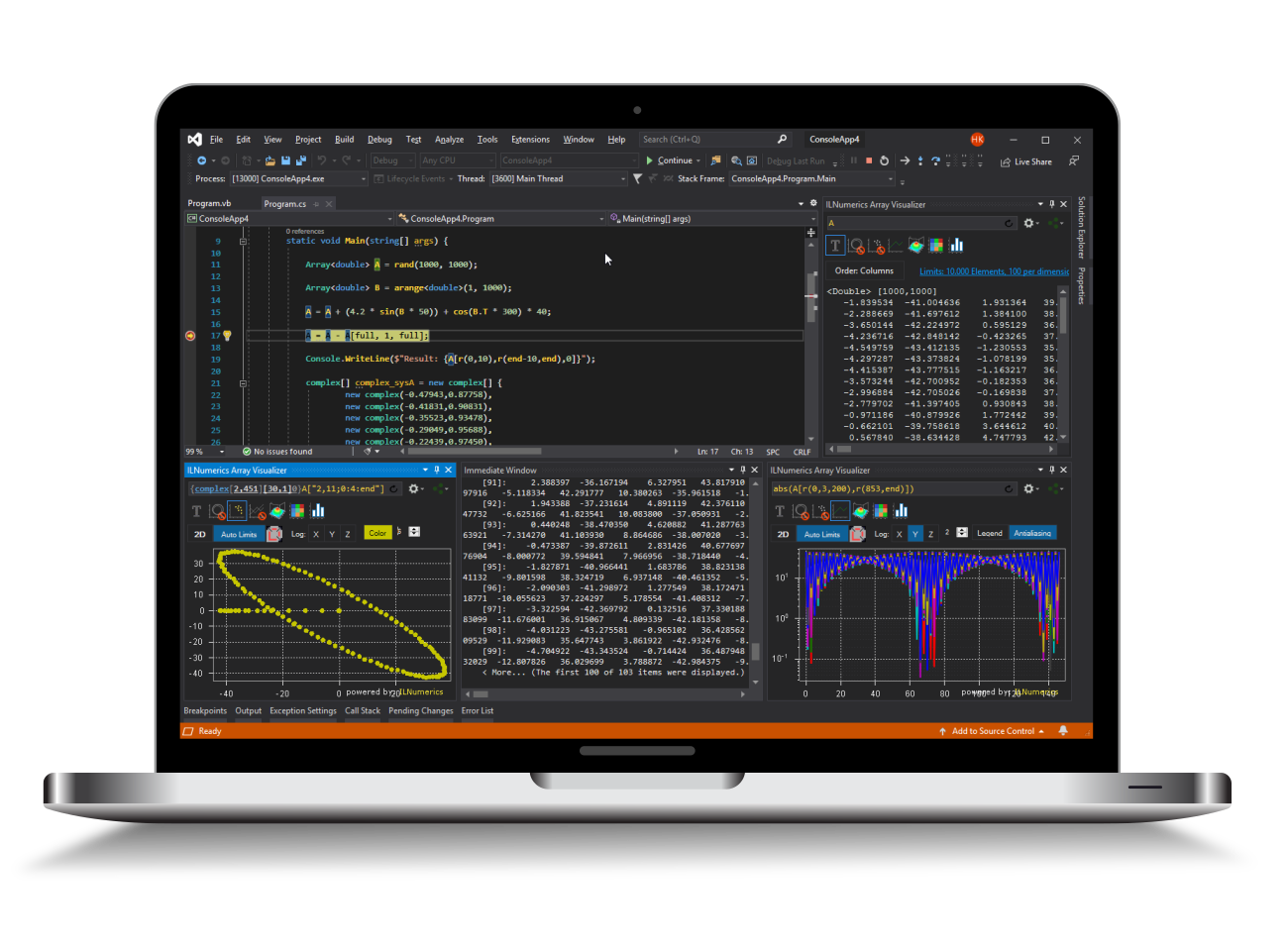Industrial Data Science
in C# and .NET:
Simple. Fast. Reliable.ILNumerics - Technical Computing

Modern High Performance Tools for Technical

Computing and Visualization in Industry and Science

tgt

# The Cholesky Decomposition in .NET (C#, Visual Basic)

With the cholesky decomposition a symmetric real or hermitian complex matrix $A$ is factorized into a lower triangular matrix $L$. It is necessary that the matrix $A$ is positive definite.

$$A = L \cdot L^*$$

where $^*$ indicates the transpose and complex conjugate.

## Using the Cholesky Decomposition in .NET (C#, Visual Basic)

If the matrix A is not positive definite L will contain a submatrix that is lower triangular.